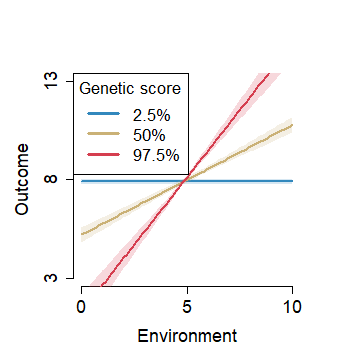*Note that you can cite this work as: *

Jolicoeur-Martineau, A., Wazana, A., Szekely, E., Steiner, M., Fleming, A. S., Kennedy, J. L., Meaney M. J. & Greenwood, C.M. (2017). Alternating optimization for GxE modelling with weighted genetic and environmental scores: examples from the MAVAN study. arXiv preprint arXiv:1703.08111.

Jolicoeur-Martineau, A., Belsky, J., Szekely, E., Widaman, K. F., Pluess, M., Greenwood, C., & Wazana, A. (2017). Distinguishing differential susceptibility, diathesis-stress and vantage sensitivity: beyond the single gene and environment model. arXiv preprint arXiv:1712.04058.

## GxE testing

From version 1.2.0 of the LEGIT package, we introduce a function to test for different types of gene-by-environment interactions (GxE) as per Belsky et al. (2013). See our preprint: https://psyarxiv.com/27uw8. We want to test if the GxE reflects diathesis stress, differential susceptibility or vantage sensitivity.

The figure below is a graphical representation of the different types of interactions assuming a STRONG model:

knitr::include_graphics("https://raw.githubusercontent.com/AlexiaJM/LEGIT/master/images/GxE_testing_strong.png")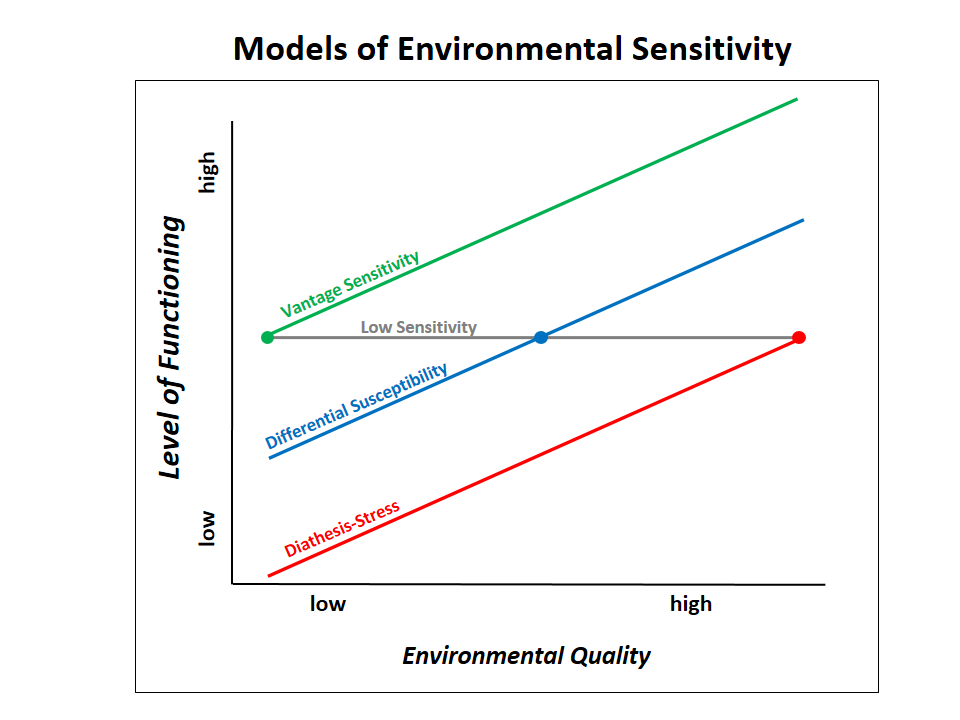The figure below is a graphical representation of the different types of interactions assuming a WEAK model:

knitr::include_graphics("https://raw.githubusercontent.com/AlexiaJM/LEGIT/master/images/GxE_testing_weak.png")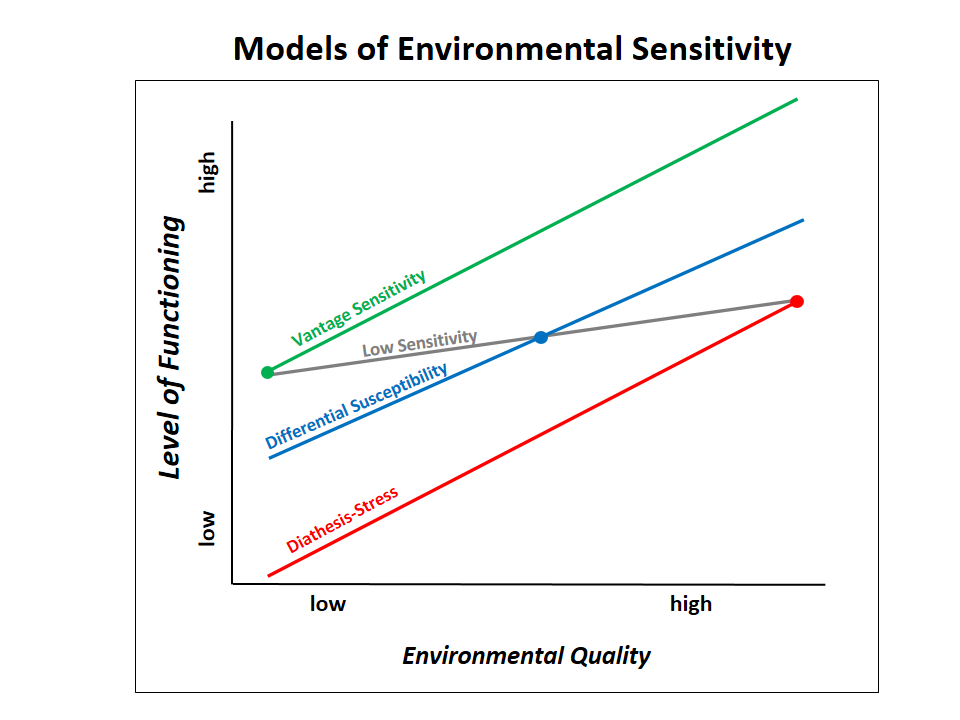To test for differential susceptibility, Widaman et al. (2013) estimate the cross-over point of the model. This can be done easily with LEGIT by simply adding a negative intercept to the environment E. This can be seen by the following: $y = 2 + 3(E-c) + 5G(E-c) \ E = q_1e_1 + q_2e_2 + … + q_le_l$ is equivalent to $y = 2 + 3E' + 5GE' \ E' = -c + q_1e_1 + q_2e_2 + … + q_le_l$

To test for vantage sensitivity and diathesis-stress, we can use the model above but fix the cross-over point to the expected minimum and maximum of $$E$$ respectively.

## Simulation of diathesis-stress, vantage sensitivity and differential susceptibility

Let's look at a two-way interaction model with a cross-over point and a continuous outcome:

$\mathbf{g}_j \sim Binomial(n=1,p=.30) \ j = 1, 2, 3, 4$ $\mathbf{e}_l \sim 10 Beta(\alpha=2,\beta=2) \ l = 1, 2, 3$ $\mathbf{g} = .30\mathbf{g}_1 + .10\mathbf{g}_2 + .20\mathbf{g}_3 + .40\mathbf{g}_4$ $\mathbf{e} = .45\mathbf{e}_1 + .35\mathbf{e}_2 + .20\mathbf{e}_3$ $\mathbf{\mu} = 3 + \beta_E(\mathbf{e}-c) + 2\mathbf{g}(\mathbf{e}-c)$ where $$c$$ and $$\beta_E$$ depend on the model assumed. $$c = 0$$ represents vantage sensitivity, $$c = 10$$ represents diathesis-stress. We set $$c=5$$ to represents differential susceptibility. $$\beta_E = 0$$ assumes a STRONG model while $$\beta_E \ne 0$$ assumes a WEAK model.

$y \sim Normal(\mu,\sigma=1)$

Note that the environmental score E is in the range [0,10].

## Example 1: Vantage sensitivity WEAK

When assuming vantage sensitivity WEAK, we let $$c=0$$ so that: $\mathbf{\mu} = 3 + (\mathbf{e}-0) + 2\mathbf{g}(\mathbf{e}-0)$

We generate N=250 observations and test all 4 possible models based on the BIC (other choices are also available: AIC, AICc, cross-validation and cross-validated AUC). It is important to not include G or E in the model formula because they will be added automatically. We start by assuming that we don't know the true minimum of the environmental score, thus we use option crossover = c(“min”,“max”) to find the minimum/maximum from the observed data (this is the default).

set.seed(777)
library(LEGIT)
ex_dia = example_with_crossover(N=250, c=0, coef_main = c(3,1,2), sigma=1, seed=7)
GxE_test_BIC = GxE_interaction_test(data=ex_dia$data, genes=ex_dia$G, env=ex_dia$E, formula_noGxE = y ~ 1, crossover = c("min","max"), criterion="BIC") GxE_test_BIC$results

##                                    BIC       crossover crossover 95%
## Vantage sensitivity WEAK           "772.27"  "0.45"    ""
## Differential susceptibility WEAK   "775.08"  "-0.4"    "( -0.73 / -0.07 )"
## Differential susceptibility STRONG "870.68"  "2.22"    "( 1.79 / 2.64 )"
## Vantage sensitivity STRONG         "930.4"   "0.44"    ""
## Diathesis-stress WEAK              "971.12"  "9.75"    ""
## Diathesis-stress STRONG            "1123.61" "9.74"    ""
## G + E only                         "1237.56" NA        ""
## G only                             "1285.07" NA        ""
## E only                             "1308.15" NA        ""
## Intercept only                     "1341.59" NA        ""
##                                    Within observable range?
## Vantage sensitivity WEAK           ""
## Differential susceptibility WEAK   "No"
## Differential susceptibility STRONG "No"
## Vantage sensitivity STRONG         ""
## Diathesis-stress WEAK              ""
## Diathesis-stress STRONG            ""
## G + E only                         ""
## G only                             ""
## E only                             ""
## Intercept only                     ""
##                                    % of observations below crossover
## Vantage sensitivity WEAK           "0"
## Differential susceptibility WEAK   "0"
## Differential susceptibility STRONG "0.004"
## Vantage sensitivity STRONG         "0"
## Diathesis-stress WEAK              "1"
## Diathesis-stress STRONG            "1"
## G + E only                         NA
## G only                             NA
## E only                             NA
## Intercept only                     NA


Here we see the results. Notice that vantage sensitivity WEAK is the model with the lowest BIC. Differential susceptibility WEAK has a slightly worse fit and the crossover point is not within observable range, thus we reject it. Note that we only show the element results of the output, but the 6 models (weak/strong vantage sensitivity, diathesis-stress and differential susceptibility) are also returned.

Considering that we know that the minimum and maximum of E are 0 and 10 respectively, we can also refit the models with crossover = c(0, 10).

GxE_test_BIC_ = GxE_interaction_test(data=ex_dia$data, genes=ex_dia$G, env=ex_dia$E, formula_noGxE = y ~ 1, crossover = c(0, 10), criterion="BIC") GxE_test_BIC_$results

##                                    BIC       crossover crossover 95%
## Vantage sensitivity WEAK           "770.07"  "0"       ""
## Differential susceptibility WEAK   "775.08"  "-0.4"    "( -0.73 / -0.07 )"
## Differential susceptibility STRONG "870.68"  "2.22"    "( 1.79 / 2.64 )"
## Vantage sensitivity STRONG         "948.33"  "0"       ""
## Diathesis-stress WEAK              "965.08"  "10"      ""
## Diathesis-stress STRONG            "1125.2"  "10"      ""
## G + E only                         "1237.56" NA        ""
## G only                             "1285.07" NA        ""
## E only                             "1308.15" NA        ""
## Intercept only                     "1341.59" NA        ""
##                                    Within observable range?
## Vantage sensitivity WEAK           ""
## Differential susceptibility WEAK   "No"
## Differential susceptibility STRONG "No"
## Vantage sensitivity STRONG         ""
## Diathesis-stress WEAK              ""
## Diathesis-stress STRONG            ""
## G + E only                         ""
## G only                             ""
## E only                             ""
## Intercept only                     ""
##                                    % of observations below crossover
## Vantage sensitivity WEAK           "0"
## Differential susceptibility WEAK   "0"
## Differential susceptibility STRONG "0.004"
## Vantage sensitivity STRONG         "0"
## Diathesis-stress WEAK              "1"
## Diathesis-stress STRONG            "1"
## G + E only                         NA
## G only                             NA
## E only                             NA
## Intercept only                     NA


We obtain similar results.

We can then plot the best model:

plot(GxE_test_BIC$fits$vantage_sensitivity_WEAK, xlim=c(0,10), ylim=c(3,13),cex.leg=1.4, cex.axis=1.5, cex.lab=1.5)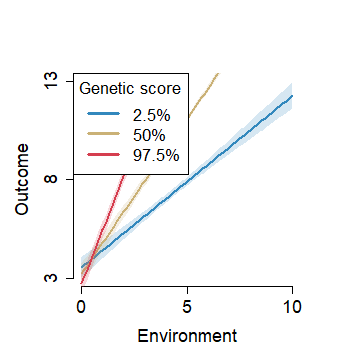## Example 2: Vantage sensitivity STRONG

When assuming vantage sensitivity STRONG, we let $$c=0$$ and $$\beta_E=0$$ so that: $\mathbf{\mu} = 3 + 2\mathbf{g}(\mathbf{e}-0)$

We generate N=250 observations and test all 6 possible models based on the BIC assuming we don't know what is the minimum environmental score.

ex_dia_s = example_with_crossover(N=250, c=0, coef_main = c(3,0,2), sigma=1, seed=7)
GxE_test_BIC = GxE_interaction_test(data=ex_dia_s$data, genes=ex_dia_s$G, env=ex_dia_s$E, formula_noGxE = y ~ 1, crossover = c("min","max"), criterion="BIC") GxE_test_BIC$results

##                                    BIC       crossover crossover 95%
## Differential susceptibility STRONG "770.17"  "-0.5"    "( -1.22 / 0.23 )"
## Vantage sensitivity STRONG         "771.77"  "0.45"    ""
## Vantage sensitivity WEAK           "772.93"  "0.45"    ""
## Differential susceptibility WEAK   "775.68"  "-0.45"   "( -1.18 / 0.27 )"
## Diathesis-stress WEAK              "967.38"  "9.74"    ""
## Diathesis-stress STRONG            "1104.84" "9.75"    ""
## G + E only                         "1170.07" NA        ""
## G only                             "1171.24" NA        ""
## Intercept only                     "1247.53" NA        ""
## E only                             "1248.73" NA        ""
##                                    Within observable range?
## Differential susceptibility STRONG "No"
## Vantage sensitivity STRONG         ""
## Vantage sensitivity WEAK           ""
## Differential susceptibility WEAK   "No"
## Diathesis-stress WEAK              ""
## Diathesis-stress STRONG            ""
## G + E only                         ""
## G only                             ""
## Intercept only                     ""
## E only                             ""
##                                    % of observations below crossover
## Differential susceptibility STRONG "0"
## Vantage sensitivity STRONG         "0"
## Vantage sensitivity WEAK           "0"
## Differential susceptibility WEAK   "0"
## Diathesis-stress WEAK              "1"
## Diathesis-stress STRONG            "1"
## G + E only                         NA
## G only                             NA
## Intercept only                     NA
## E only                             NA


We conclude that vantage sensitivity STRONG is the best model.

We can then plot the best model:

plot(GxE_test_BIC$fits$vantage_sensitivity_STRONG, xlim=c(0,10), ylim=c(3,13),cex.leg=1.4, cex.axis=1.5, cex.lab=1.5)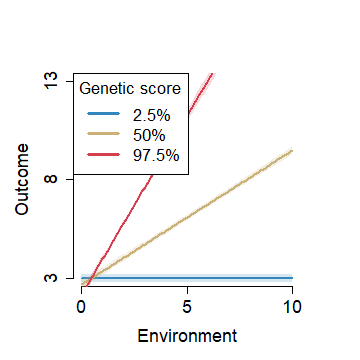## Example 3: Differential susceptibility WEAK

When assuming differential susceptibility WEAK, we let $$c=5$$ (and use 8 instead of 3 as intercept to keep a similar outcome range) so that: $\mathbf{\mu} = 8 + (\mathbf{e}-5) + 2\mathbf{g}(\mathbf{e}-5)$

We generate N=250 observations and test all 6 possible models based on the BIC assuming we don't know what is the minimum environmental score.

ex_ds = example_with_crossover(N=250, c=5, coef_main = c(3+5,1,2), sigma=1, seed=7)
GxE_test_BIC = GxE_interaction_test(data=ex_ds$data, genes=ex_ds$G, env=ex_ds$E, formula_noGxE = y ~ 1, crossover = c("min","max"), criterion="BIC") GxE_test_BIC$results

##                                    BIC       crossover crossover 95%
## Differential susceptibility WEAK   "776.06"  "4.83"    "( 4.5 / 5.16 )"
## Diathesis-stress WEAK              "834.53"  "9.74"    ""
## Vantage sensitivity WEAK           "835.05"  "0.45"    ""
## Differential susceptibility STRONG "864.07"  "4.78"    "( 4.37 / 5.2 )"
## E only                             "1011.14" NA        ""
## G + E only                         "1011.79" NA        ""
## Intercept only                     "1149.96" NA        ""
## G only                             "1153.2"  NA        ""
## Diathesis-stress STRONG            "1157.61" "6.95"    ""
## Vantage sensitivity STRONG         "1159.69" "-2.28"   ""
##                                    Within observable range?
## Differential susceptibility WEAK   "Yes"
## Diathesis-stress WEAK              ""
## Vantage sensitivity WEAK           ""
## Differential susceptibility STRONG "Yes"
## E only                             ""
## G + E only                         ""
## Intercept only                     ""
## G only                             ""
## Diathesis-stress STRONG            ""
## Vantage sensitivity STRONG         ""
##                                    % of observations below crossover
## Differential susceptibility WEAK   "0.488"
## Diathesis-stress WEAK              "1"
## Vantage sensitivity WEAK           "0"
## Differential susceptibility STRONG "0.476"
## E only                             NA
## G + E only                         NA
## Intercept only                     NA
## G only                             NA
## Diathesis-stress STRONG            "1"
## Vantage sensitivity STRONG         "0.044"


We conclude that differential susceptibility WEAK is the best model.

We can then plot the best model:

plot(GxE_test_BIC$fits$diff_suscept_WEAK, xlim=c(0,10), ylim=c(3,13),cex.leg=1.4, cex.axis=1.5, cex.lab=1.5)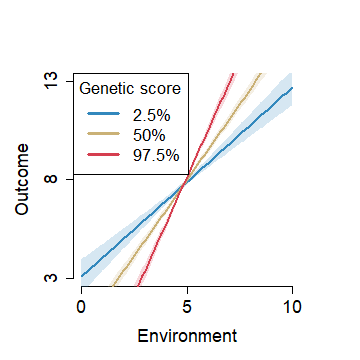## Example 4: Differential susceptibility STRONG

When assuming differential susceptibility STRONG, we let $$c=5$$ and $$\beta_E=0$$ (and use 8 instead of 3 as intercept to keep a similar outcome range) so that: $\mathbf{\mu} = 8 + 2\mathbf{g}(\mathbf{e}-5)$

We generate N=250 observations and test all 6 possible models based on the BIC assuming we don't know what is the minimum environmental score.

ex_ds_s = example_with_crossover(N=250, c=5, coef_main = c(3+5,0,2), sigma=1, seed=7)
GxE_test_BIC = GxE_interaction_test(data=ex_ds_s$data, genes=ex_ds_s$G, env=ex_ds_s$E, formula_noGxE = y ~ 1, crossover = c("min","max"), criterion="BIC") GxE_test_BIC$results

##                                    BIC      crossover crossover 95%
## Differential susceptibility STRONG "771.2"  "4.84"    "( 4.13 / 5.55 )"
## Differential susceptibility WEAK   "776.64" "4.84"    "( 4.12 / 5.55 )"
## Diathesis-stress WEAK              "834.4"  "9.74"    ""
## Vantage sensitivity WEAK           "834.9"  "0.44"    ""
## E only                             "848.02" NA        ""
## G + E only                         "851.11" NA        ""
## Intercept only                     "895.99" NA        ""
## G only                             "899.81" NA        ""
## Diathesis-stress STRONG            "909.24" "6.77"    ""
## Vantage sensitivity STRONG         "913.1"  "-2.45"   ""
##                                    Within observable range?
## Differential susceptibility STRONG "Yes"
## Differential susceptibility WEAK   "Yes"
## Diathesis-stress WEAK              ""
## Vantage sensitivity WEAK           ""
## E only                             ""
## G + E only                         ""
## Intercept only                     ""
## G only                             ""
## Diathesis-stress STRONG            ""
## Vantage sensitivity STRONG         ""
##                                    % of observations below crossover
## Differential susceptibility STRONG "0.484"
## Differential susceptibility WEAK   "0.484"
## Diathesis-stress WEAK              "1"
## Vantage sensitivity WEAK           "0"
## E only                             NA
## G + E only                         NA
## Intercept only                     NA
## G only                             NA
## Diathesis-stress STRONG            "1"
## Vantage sensitivity STRONG         "0.036"


We conclude that differential susceptibility STRONG is the best model.

We can then plot the best model:

plot(GxE_test_BIC$fits$diff_suscept_STRONG, xlim=c(0,10), ylim=c(3,13),cex.leg=1.4, cex.axis=1.5, cex.lab=1.5)